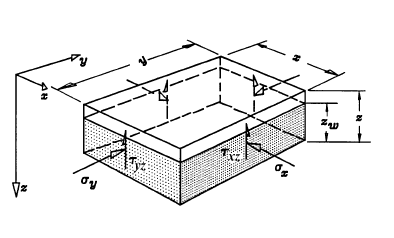The Rocscience International Conference 2021 Proceedings are now available. Read Now

Lateral Stress Overview

Lateral Stress toggles between two options, Gravity and Constant.

• With the Gravity option, the user inputs locked in stress (lateral stress at the pillar surface) and horizontal / vertical stress ratios, from which the lateral stresses are calculated.
• With the Constant option, the user inputs the actual lateral effective stresses.

The corresponding options in the input menu will change as this option is toggled.

Rectangular Pillar

For a rectangular pillar, the lateral stresses are always applied normal to the abutments (in the x and y directions). Therefore, the effective lateral stress = effective normal stress on the abutments.

Relationship between stress directions and pillar dimensionsPolygonal Pillar

For a polygonal pillar, the lateral stresses are not always applied normal to the abutments since the polygon segments can be oriented arbitrarily. In addition, the abutments may be non-vertical.

The horizontal principal stresses, Sigma 1 and Sigma 3, are always horizontal and perpendicular to one another. The rotation of the horizontal principal stresses in the x-y plane is dictated by Trend 3 (measured clockwise from the y-axis (or North)).

A 2D stress transformation is applied to the horizontal principal stresses in order to obtain the horizontal stresses in the global x-y coordinates. For the case of non-vertical abutments, the vertical stress also impacts the normal stresses on the abutments. The vertical stress is calculated by the effective weight of everything above the midway height of the pillar. The normal stress takes into account the components of all the principle stresses which act normal to the abutment face.

Finally, of the height of water is greater than the (pillar + overburden) thickness, the difference will appear as free water above the pillar. These situations are illustrated above.

Water Height

Note that the Water Height is measured from the bottom of the pillar, whereas the pillar and overburden thicknesses are entered as absolute values. This is because water pressure is taken into account if the pillar is specified as "permeable" (RIGID and ELASTIC options only) therefore water heights less than the pillar thickness can be specified to account for the effect of water pressure on effective lateral stress.

If a water height less than the pillar + overburden thickness is specified, the user will notice a line representing the water height, drawn on the model at the appropriate level. Any water above the pillar + overburden is referred to as free water.

Overburden Thickness

A thickness of overburden can be added above the pillar. This is the absolute value of the overburden thickness, and is NOT measured from the base of the pillar, as is the water height.

Locked In Stress

This is the lateral stress at the surface of the pillar. This value can be manipulated to represent preexisting stress conditions, or the effects of the overburden on the lateral stress.

Horizontal to Vertical Stress Ratios

Used with the Gravity lateral stress option.

Horizontal Effective Stresses

Used with the Constant lateral stress option.